Maple Tutorial for Linear Algebra

Introduction

On most platforms, Maple V is started by double-clicking on the Maple icon. However, in our Computer Lab in HH-3030/3056 you can simply type xmaple or xmaple & to start a Maple session. If you are familiar with the Unix environment you can create a Maple subdirectory and start Maple from within that subdirectory.

We will introduce Maple commands which will be useful in Mathematics 2050 or 2051. Maple V provides a wide range of special packages specially designed for students. For example, there are packages for calculus, statistics, number theory, group theory, and graph theory. One of the most frequently used packages is the linear algebra package, linalg . This package provides a complete set of commands for working with vectors and matrices.

First load the linear algebra package using the with command. If you do not wish to load the entire package, you can load selected routines from the package using the syntax

with(packagename, routine1, ..., routineN);

You can also use the long form of the routine name

packagename[routinename](...);

> with(linalg);

`Warning, new definition for norm`

`Warning, new definition for trace`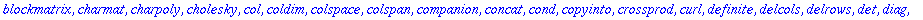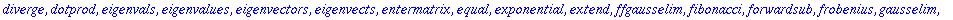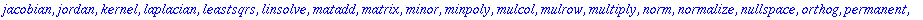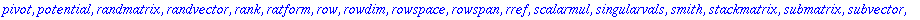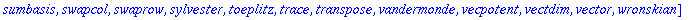Note the many functions that are listed above. To find more information on any topic you can look at the help pages by typing ?topic . For example, ?addrow will show you clearly, with examples, how to use this function. Experiment, by executing the command ?addrow on a command line.

Solving Systems of Equations

An array is a generalization of the matrix data structure. The conversion between arrays and matrices is automatic, so following the creation of an array it can be used as a matrix.

Consider the 4x6 numeric matrix created using the array command.

> A:=array([[1,3,-2,0,2,0],[2,6,-5,-2,4,-3],[0,0,5,10,0,15],[2,6,0,8,0,18]]);The above label A always refers to the above matrix unless we unassign the symbol A. Note the use of the semicolin (;). The semicolin is always needed in order to see the output. If you do not want to see the output, end the command with the colin (:) instead.

> A;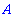The matrix is not displayed. It is displayed using the evalm command.

> evalm(A);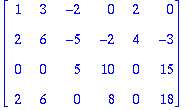The command print(A) will also work. The following command unassigns the symbol A.

> A:='A';> evalm(A);The above matrix can also be defined with the matrix command.

> A:=matrix(4,6,[1,3,-2,0,2,0,2,6,-5,-2,4,-3,0,0,5,10,0,15,2,6,0,8,0,18]);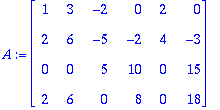> B:=vector([0,-1,5,6]);If A is the coefficient matrix and B the constant matrix of a system of 4 equations in 6 unknowns, then one way to solve the system is to form the augmented matrix.

> A_B:=augment(A,B);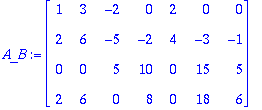Next we can row-reduce the matrix by using the commands addrow, mulrow, and swaprow , as many times as is necessary. The symbol (%) always refers to the latest output.> mulrow(%,2,-1);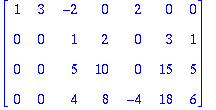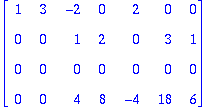> swaprow(%,3,4);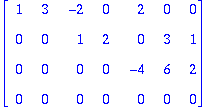> row_esc_form:=mulrow(%,3,-1/4);We could have gotten to the same place in the computation by using the gausselim command, except that the leading numbers are not necessarily 1's.

> gausselim(A_B);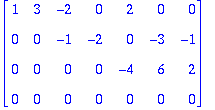To put the matrix row_esc_form in reduced row eschelon form, we continue with the following computations.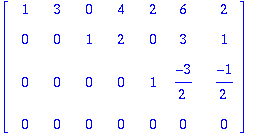As above, we can use one command in Maple to do the same thing. Either use the command rref or the following.

> gaussjord(A_B);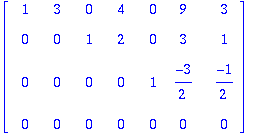As you may have already guessed, Maple has one command to do all of the above at once.

> linsolve(A,B);Note the three free variables,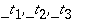. Another way to solve the system is to write the equations out, and use the solve command.

> eq1:=x1+3*x2-2*x3+2*x5=0;> eq2:=2*x1+6*x2-5*x3-2*x4+4*x5-3*x6=-1;> eq3:=5*x3+10*x4+15*x6=5;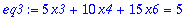> eq4:=2*x1+6*x2+8*x4+18*x6=6;> solve({eq1,eq2,eq3,eq4},{x1,x2,x3,x4,x5,x6});The free variables here are x2, x4, and x6. Show that the two solutions are equivalent. Another facility built into linalg can extract the coefficient matrix and the constant matrix from a system of linear equations.

> A:=genmatrix([eq1,eq2,eq3,eq4],[x1,x2,x3,x4,x5,x6],'B');> evalm(B);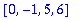Matrix Algebra

> A:=matrix(3,3,a);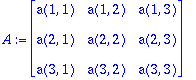> B:=matrix(3,3,b);> A+B;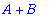> evalm(A+B);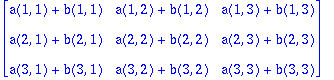> evalm(k*A);> evalm(-A);> transpose(A);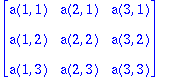> A&*B;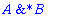> evalm(%);How can we denote the identity matrix? We cannot use the symbol I , since it is reserved for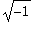. Check the help page on the word alias .

> alias(Id=&*());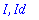> zero:=matrix(3,3,0);> evalm(zero+Id);> evalm(A^2);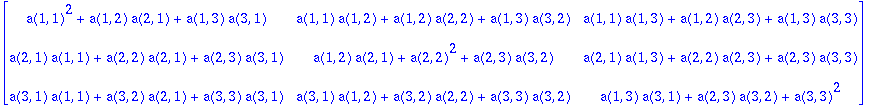> A:=matrix(2,2,[a,b,c,d]);> inverse(A);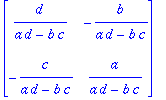> A:=matrix(3,3,[1,-2,2,2,1,1,1,0,1]);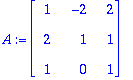> evalm(A^(-1));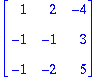> evalm(%%&*%); # Note (%%) refers to second last output. The stuff after the symbol # is a comment and is not executed on the command line.